Courses

# Test: Alligation Or Mixture- 1

## 10 Questions MCQ Test General Aptitude for GATE 2020 | Test: Alligation Or Mixture- 1

Description
This mock test of Test: Alligation Or Mixture- 1 for Quant helps you for every Quant entrance exam. This contains 10 Multiple Choice Questions for Quant Test: Alligation Or Mixture- 1 (mcq) to study with solutions a complete question bank. The solved questions answers in this Test: Alligation Or Mixture- 1 quiz give you a good mix of easy questions and tough questions. Quant students definitely take this Test: Alligation Or Mixture- 1 exercise for a better result in the exam. You can find other Test: Alligation Or Mixture- 1 extra questions, long questions & short questions for Quant on EduRev as well by searching above.
QUESTION: 1

### Practice Quizzes or MCQ (Multiple Choice Questions) with solution are available for Practice, which would help you prepare for Alligation or Mixture under Quantitative Aptitude. You can practice these practice quizzes as per your speed and improvise the topic. The same topic is covered under various competitive examinations like - CAT, GMAT, Bank PO, SSC and other competitive examinations. Q. A container contains 40 litres of milk. From this container 4 litres of milk was taken out and replaced by water. This process was repeated further two times. How much milk is now contained by the container?

Solution:

Assume that a container contains x of liquid from which y units are taken out and replaced by water. After n operations, the quantity of pure liquid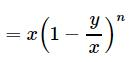Hence milk now contained by the container = 40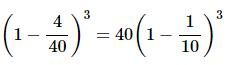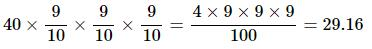QUESTION: 2

### 8 litres are drawn from a cask full of wine and is then filled with water. This operation is performed three more times. The ratio of the quantity of wine now left in cask to that of the water is 16:81. How much wine did the cask originally hold?

Solution:

Let the quantity of the wine in the cask originally be x litres.

Then, quantity of wine left in the cask after 4 operations = [x(1 − 8/x)4] litres.

[x(1 − 8/x)4]/x = 16/81

(x - 8/x)4 = (2/3)4

= 3x - 24 = 2x

x = 24

QUESTION: 3

### How many kilograms of sugar costing Rs. 9 per kg must be mixed with 27 kg of sugar costing Rs. 7 per Kg so that there may be a gain of 10 % by selling the mixture at Rs. 9.24 per Kg ?

Solution:

S.P. of 1 kg of mixture = Rs. 9.24, Gain 10%.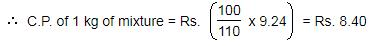By the rule of allilation, we have: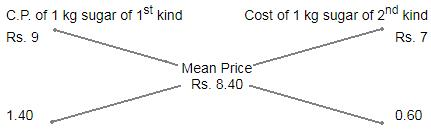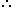Ratio of quantities of 1st and 2nd kind = 14 : 6 = 7 : 3.

Let x kg of sugar of 1st be mixed with 27 kg of 2nd kind.

Then, 7 : 3 = x : 27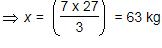QUESTION: 4

A milk vendor has 2 cans of milk. The first contains 25% water and the rest milk. The second contains 50% water. How much milk should he mix from each of the containers so as to get 12 litres of milk such that the ratio of water to milk is 3 : 5?

Solution:

The correct option is C.

Let x and (12-x) litres of milk be mixed from the first and second container respectively

Amount of milk in x litres of the the first container = .75x

Amount of water in x litres of the the first container = .25x

Amount of milk in (12-x) litres of the the second container = .5(12-x)

Amount of water in (12-x) litres of the the second container = .5(12-x)

Ratio of water to milk = [.25x + .5(12-x)] : [.75x + .5(12-x)] = 3 : 5

⇒ (.25x+6-5x)/(.75x+6-.5x) =3/5

⇒(6−.25x)/(.25x+6) =3/5

⇒30−1.25x=.75x+18

⇒2x=12

⇒x=6

Since x = 6, 12-x = 12-6 = 6

Hence 6 and 6 litres of milk should mixed from the first and second container respectively

QUESTION: 5

Tea worth Rs. 126 per kg and Rs. 135 per kg are mixed with a third variety of tea in the ratio 1 : 1 : 2. If the mixture is worth Rs. 153 per kg, what is the price of the third variety per kg ?

Solution:

Since first and second varieties are mixed in equal proportions.So, their average price = Rs.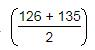= Rs. 130.50

So, the mixture is formed by mixing two varieties, one at Rs. 130.50 per kg and the other at say, Rs. x per kg in the ratio 2 : 2, i.e., 1 : 1. We have to find x.

By the rule of alligation, we have: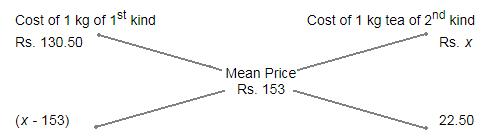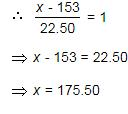QUESTION: 6

Two vessels A and B contain spirit and water in the ratio 5 : 2 and 7 : 6 respectively. Find the ratio in which these mixture be mixed to obtain a new mixture in vessel C containing spirit and water in the ration 8 : 5 ?

Solution:

Spirit in 1 litre mix of A = 5/7 litre.
Spirit in 1 litre mix of B = 7/13 litre.
Spirit in 1 litre mix of C = 8/13 litre.
By rule of alligation we have required ratio X:Y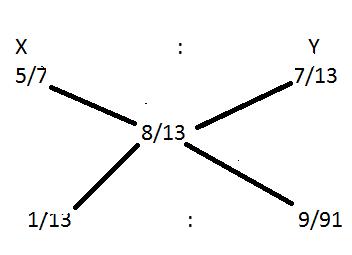Therefore required ratio = 1/13 : 9/91
= 7:9

QUESTION: 7

A jar is full of whiskey contains 40% alcohol. A part of this whiskey is replaced by another containing 19% alcohols and now the percentage of alcohol was found to be 26%. The quantity of whiskey replaced is:

Solution:

By the rule of alligation we have
So ratio of 1st and 2nd quantities = 7 : 14 = 1 : 2
∴ Required quantity replaced = 2/3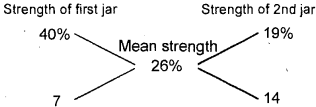QUESTION: 8

In what ratio should rice at Rs.9.30 per Kg be mixed with rice at Rs. 10.80 per Kg so that the mixture be worth Rs.10 per Kg?

Solution: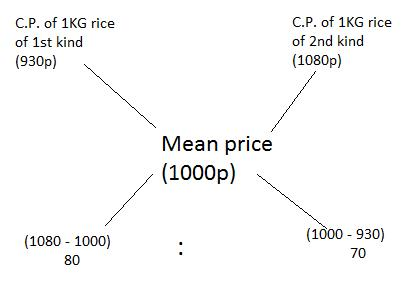Thus, required ratio = 80 : 70 = 8 : 7

QUESTION: 9

Find the ratio in which rice at Rs. 7.20 a kg be mixed with rice at Rs. 5.70 a kg to produce a mixture worth Rs. 6.30 a kg.

Solution: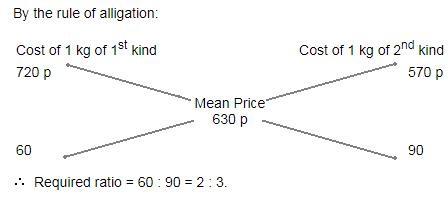QUESTION: 10

The cost of Type 1 material is Rs. 15 per kg and Type 2 material is Rs.20 per kg. If both Type 1 and Type 2 are mixed in the ratio of 2 : 3, then what is the price per kg of the mixed variety of material?

Solution:

Cost Price(CP) of Type 1 material is Rs. 15 per kg
Cost Price(CP) of Type 2 material is Rs. 20 per kg
Type 1 and Type 2 are mixed in the ratio of 2 : 3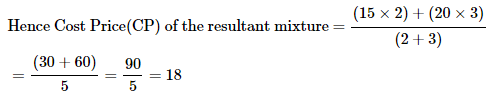Therefore, rice per kg of the mixed variety of material = Rs.18